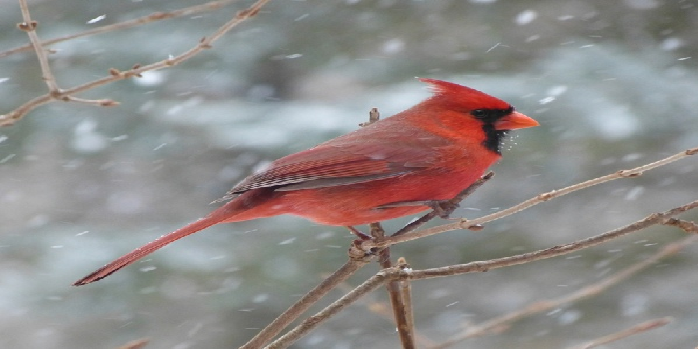# How to load an image and show the image using Keras?

MatplotlibPythonData Visualization

#### Python Data Science basics with Numpy, Pandas and Matplotlib

Most Popular

63 Lectures 6 hours

#### Data Visualization using MatPlotLib & Seaborn

11 Lectures 4 hours

#### MatPlotLib with Python

9 Lectures 2.5 hours

To load an image and show the image using Keras, we will use load_image() method to load an image and set the target size of the image to be shown.

## Steps

• Use load_img() method to load the figure.
• Set the target size of the image.
• To display the figure, use show() method.

## Example

from keras.preprocessing import image
img = image.load_img('bird.jpg', target_size=(350, 750))

img.show()

## Output